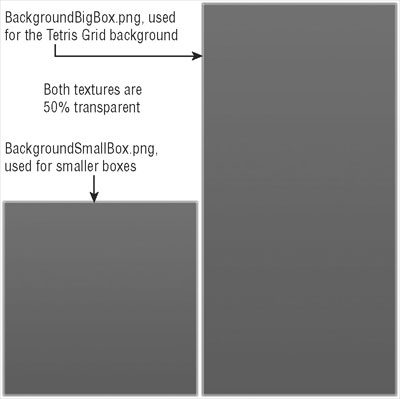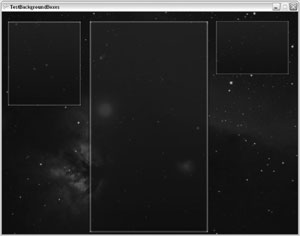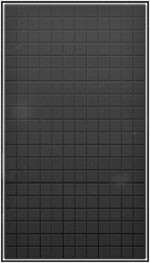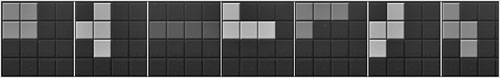# §4.4俄罗斯方块## 渲染背景（Rendering the Background）

public static void TestBackgroundBoxes()

{

TestGame.Start("TestBackgroundBoxes",delegate{

// Render background

TestGame.game.background.Render();

// Draw background boxes for all the components

TestGame.game.backgroundBigBox.Render(new Rectangle((512 - 200) - 15, 40 - 12, 400 + 23, (768 - 40) + 16));

TestGame.game.backgroundSmallBox.Render(new Rectangle((512 - 480) - 15, 40 - 10, 290 - 30, 300));

TestGame.game.backgroundSmallBox.Render(new Rectangle((512 + 240) - 15, 40 - 10, 290 - 30, 190)); } );

} // TestBackgroundBoxes()## 处理Grid网格（Handling the Grid）

#region Constants

public const int GridWidth = 12;

public const int GridHeight = 20;

// Calc sizes for block, etc.

int blockWidth = gridRect.Width/GridWidth;

int blockHeight = gridRect.Height/GridHeight;

for ( int x=0; x<GridWidth; x++ )

for ( int y=0; y<GridHeight; y++ )

{

game.BlockSprite.Render(new Rectangle(gridRect.X + x * blockWidth,gridRect.Y + y * blockHeight,blockWidth-1, blockHeight-1),new Color(60, 60, 60, 128)); // Empty color

} // for for

gridRect变量被作为一个参数从主类中被传递给Draw方法，以指定你想绘制grid的区域。它和你用在背景盒的矩形一样，只是稍小一点以适应。你在这里要做的第一件事就是计算每个将要绘制的砖块的宽度和高度。然后遍历整个数组，借助于SpriteHelper.Render方法使用一种半透明的暗色绘制每个砖块来展示一个空的grid场地。如图 4-9所示可以看到看上去什么样子。因为你实际上使用游戏组件你也无须在单元测试中作所有的代码。单元测试仅仅绘制背景盒，然后调用TetrisGrid.Draw 方法来显示结果（见TestEmptyGrid 单元测试）## Block类型（Block Types）/// <summary>

/// Block types we can have for each new block that falls down.

/// </summary>

/// public enum BlockTypes {

Empty, Block, Triangle, Line, RightT, LeftT, RightShape, LeftShape,

} // enum BlockTypes

/// <summary>

/// Number of block types we can use for each grid block.

/// </summary>

/// <summary>

/// Block colors for each block type.

/// </summary>

public static readonly Color[] BlockColor = new Color[] {

new Color( 60, 60, 60, 128 ), // Empty, color unused

new Color( 50, 50, 255, 255 ), // Line, blue

new Color( 160, 160, 160, 255 ), // Block, gray

new Color( 255, 50, 50, 255 ), // RightT, red

new Color( 255, 255, 50, 255 ), // LeftT, yellow

new Color( 50, 255, 255, 255 ), // RightShape, teal

new Color( 255, 50, 255, 255 ), // LeftShape, purple

new Color( 50, 255, 50, 255 ), // Triangle, green

}; // Color[] BlockColor

/// <summary>

/// Unrotated shapes

/// </summary>

public static readonly int[][,] BlockTypeShapesNormal = new int[][,] {

// Empty

new int[,] { { 0 } },

// Line

new int[,] { { 0, 1, 0 }, { 0, 1, 0 }, { 0, 1, 0 }, { 0, 1, 0 } },

// Block

new int[,] { { 1, 1 }, { 1, 1 } },

// RightT

new int[,] { { 1, 1 }, { 1, 0 }, { 1, 0 } },

// Left

new int[,] { { 1, 1 }, { 0, 1 }, { 0, 1 } },

// RightShape

new int[,] { { 0, 1, 1 }, { 1, 1, 0 } },

// LeftShape

new int[,] { { 1, 1, 0 }, { 0, 1, 1 } },

// Triangle

new int[,] { { 0, 1, 0 }, { 1, 1, 1 }, { 0, 0, 0 } },

}; // BlockTypeShapesNormal

BlockTypes 是我们谈到过的enum枚举类型；它包含所有可能的砖块类型，也在NextBlock游戏组件中被用于随机生成新砖块。最初的所有grid区域被空的砖块类型所填充。grid被定义为：

/// <summary>

/// The actual grid, contains all blocks,

/// including the currently falling block.

/// </summary>

BlockTypes[,] grid = new BlockTypes[GridWidth, GridHeight];

BlockColor[(int)grid[x,y]]

// Randomize block type and rotation

currentBlockType = (int)nextBlock.SetNewRandomBlock();

currentBlockRot = RandomHelper.GetRandomInt(4);

// Get precalculated shape

int[,] shape = BlockTypeShapes[currentBlockType,currentBlockRot];

int xPos = GridWidth/2-shape.GetLength(0)/2;

// Center block at top most position of our grid

currentBlockPos = new Point(xPos, 0);

for ( int x=0; x<shape.GetLength(0); x++ )

for ( int y=0; y<shape.GetLength(1); y++ )

if ( shape[x,y] > 0 )

{

// Check if there is already something

if (grid[x + xPos, y] != BlockTypes.Empty)

{

// Then game is over dude!

gameOver = true;

Sound.Play(Sound.Sounds.Lose);

} // if

else {

grid[x + xPos, y] = (BlockTypes)currentBlockType;

floatingGrid[x + xPos, y] = true; } // else

} // for for if

## 重力（Gravity）

// Try to move floating stuff down

if (MoveBlock(MoveTypes.Down) == false ||movingDownWasBlocked)

{

// Failed? Then fix floating stuff, not longer moveable!

for ( int x=0; x<GridWidth; x++ )

for ( int y=0; y<GridHeight; y++ )

floatingGrid[x,y] = false;

Sound.Play(Sound.Sounds.BlockFalldown);

} // if

movingDownWasBlocked = false;

// Check if we got any moveable stuff,

// if not add new random block at top!

bool canMove = false;

for ( int x=0; x<GridWidth; x++ )

for ( int y=0; y<GridHeight; y++ )

if ( floatingGrid[x,y] ) canMove = true;

if (canMove == false)

{

int linesKilled = 0;

// Check if we got a full line

for ( int y=0; y<GridHeight; y++ )

{

bool fullLine = true;

for ( int x=0; x<GridWidth; x++ )

if ( grid[x,y] == BlockTypes.Empty )

{

fullLine = false;

break;

} // for if

// We got a full line?

if (fullLine) {

// Move everything down

for ( int yDown=y-1; yDown>0; yDown  )

for ( int x=0; x<GridWidth; x++ )

grid[x,yDown+1] = grid[x,yDown];

// Clear top line

for ( int x=0; x<GridWidth; x++ )

grid[0,x] = BlockTypes.Empty;

// Add 10 points and count line

score += 10;

lines++;

linesKilled++;

Sound.Play(Sound.Sounds.LineKill);

} // if

} // for

// If we killed 2 or more lines, add extra score

if (linesKilled >= 2)

score += 5;

if (linesKilled >= 3)

score += 10;

if (linesKilled >= 4)

score += 25;

// Add new block at top

} // if

## 处理Input输入（Handling Input）

#region Move block

public enum MoveTypes {

Left,Right,Down,

} // enum MoveTypes

/// <summary>

/// Remember if moving down was blocked, this increases

/// the game speed because we can force the next block!

///</summary>

public bool movingDownWasBlocked = false;

/// <summary>

/// Move current floating block to left, right or down.

/// If anything is blocking, moving is not possible and

/// nothing gets changed!

/// </summary>

/// <returns>Returns true if moving was successful, otherwise false</returns>

public bool MoveBlock(MoveTypes moveType)

{

// Clear old pos

for ( int x=0; x<GridWidth; x++ )

for ( int y=0; y<GridHeight; y++ )

if ( floatingGrid[x,y] ) grid[x,y] = BlockTypes.Empty;

// Move stuff to new position

bool anythingBlocking = false;

Point[] newPos = new Point; int newPosNum = 0;

if ( moveType == MoveTypes.Left )

{

for ( int x=0; x<GridWidth; x++ )

for ( int y=0; y<GridHeight; y++ )

if ( floatingGrid[x,y] ) { if ( x-1 < 0 ||grid[x-1,y] != BlockTypes.Empty )

anythingBlocking = true;

else if ( newPosNum < 4 )

{

newPos[newPosNum] = new Point( x-1, y  } // else if

} // for for if

} // if (left)

else if ( moveType == MoveTypes.Right )

{

for ( int x=0; x<GridWidth; x++ )

for ( int y=0; y<GridHeight; y++ )

if ( floatingGrid[x,y] ) {

if ( x+1 >= GridWidth ||grid[x+1,y] != BlockTypes.Empty )

anythingBlocking = true;

else if ( newPosNum < 4 ) ue;

else if ( newPosNum < 4 )

{

newPos[newPosNum] = new Point( x+1, y );

newPosNum++;

} // else if

} // for for if

} // if (right)

else if ( moveType == MoveTypes.Down )

{

for ( int x=0; x<GridWidth; x++ )

for ( int y=0; y<GridHeight; y++ )

if ( floatingGrid[x,y] ) {

if ( y+1 >= GridHeight ||grid[x,y+1] != BlockTypes.Empty )

anythingBlocking = true;

else if ( newPosNum < 4 )

{

newPos[newPosNum] = new Point( x, y+1 );

newPosNum++;

} // else if

} // for for if

if ( anythingBlocking == true )

movingDownWasBlocked = true;

} // if (down)

// If anything is blocking restore old state

if ( anythingBlocking ||// Or we didn't get all 4 new positions?newPosNum != 4 )

{

for ( int x=0; x<GridWidth; x++ )

for ( int y=0; y<GridHeight; y++ )

if ( floatingGrid[x,y] ) grid[x,y] = (BlockTypes)currentBlockType;

return false;

} // if

else

{

if ( moveType == MoveTypes.Left )

currentBlockPos =new Point( currentBlockPos.X-1, currentBlockPos.Y );

else if ( moveType == MoveTypes.Right )

currentBlockPos =new Point( currentBlockPos.X+1, currentBlockPos.Y );

else if ( moveType == MoveTypes.Down )

currentBlockPos =new Point( currentBlockPos.X, currentBlockPos.Y+1 );

// Else we can move to the new position, lets do it!

for ( int x=0; x<GridWidth; x++ )

for ( int y=0; y<GridHeight; y++ )

floatingGrid[x,y] = false;

for ( int i=0; i<4; i++ )

{

grid[newPos[i].X,newPos[i].Y] = (BlockTypes)currentBlockType;

floatingGrid[newPos[i].X,newPos[i].Y] = true;

} // for

Sound.Play(Sound.Sounds.BlockMove);

return true; }

// else

}

// MoveBlock(moveType)

#endregion

## 测试

TestRotatingBlock，它测试TetrisGrid类的RotateBlock方法。

TestFallingBlockAndKillLine，它被用来测试gravity重力和你刚刚所学的用户输入。

2006 - 2023，推荐分辨率1024*768以上，推荐浏览器Chrome、Edge等现代浏览器，截止2021年12月5日的访问次数：1872万9823 站长邮箱